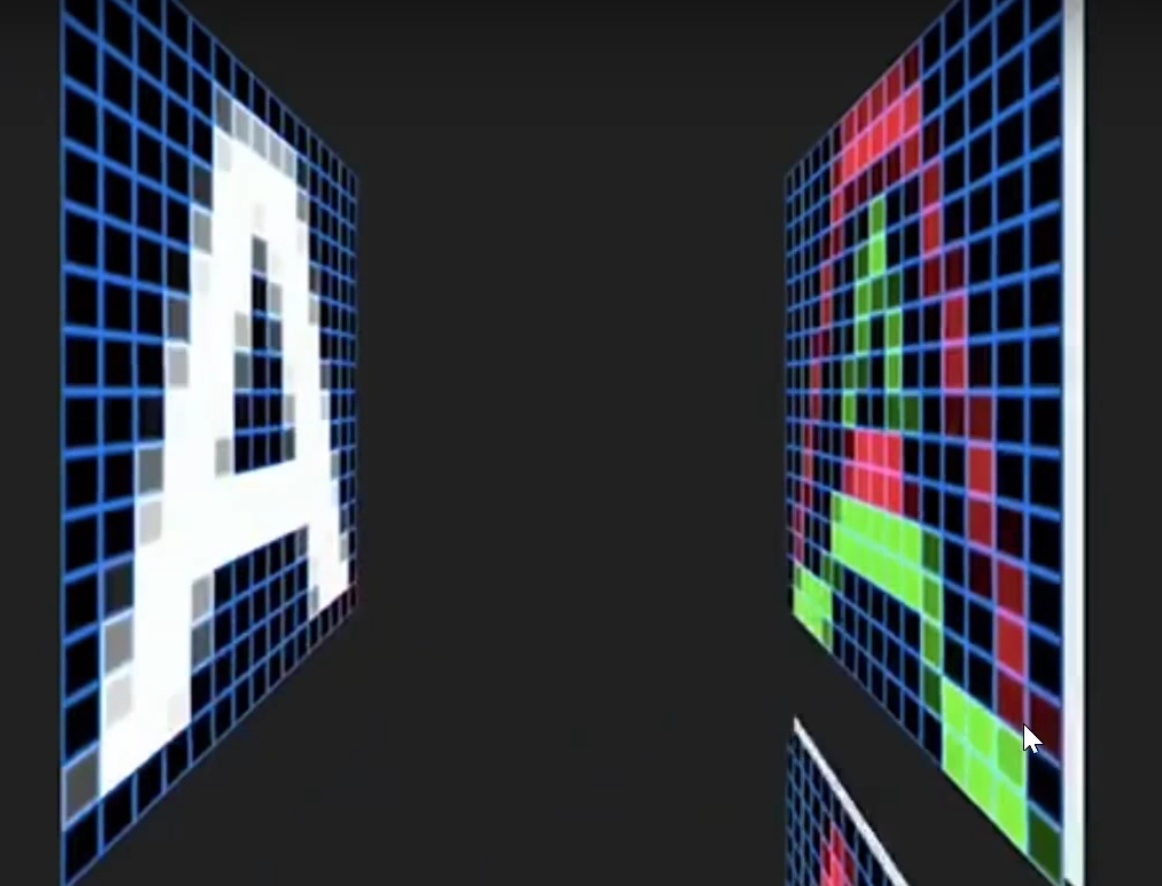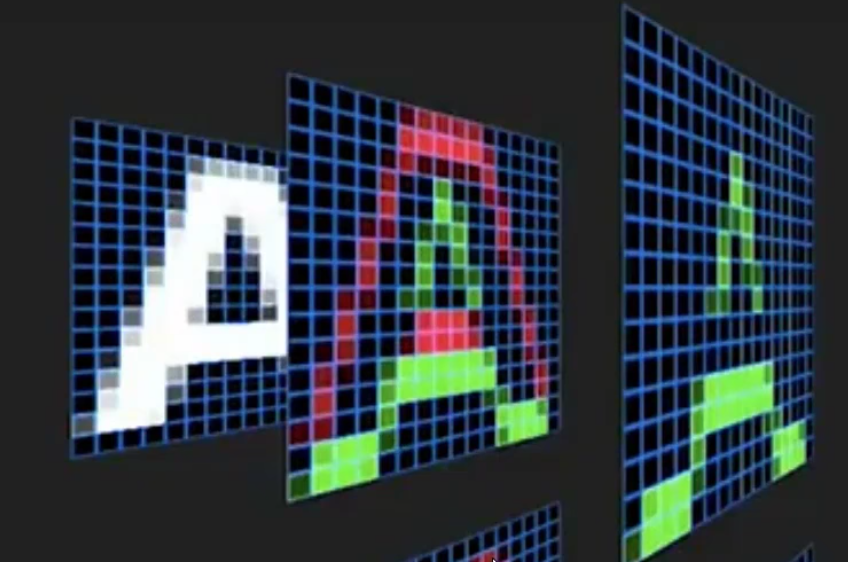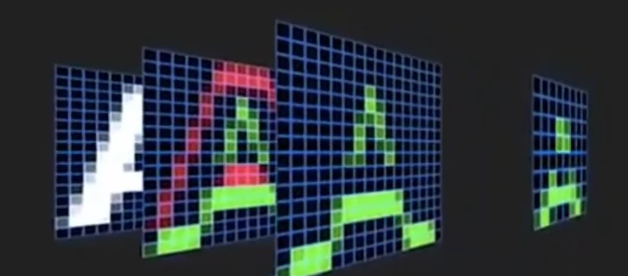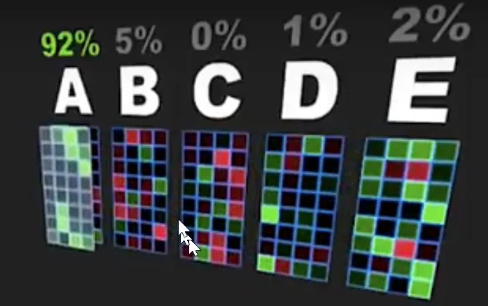# Notes for: Fast.ai 2018 - Lesson 3: Understanding convolutions

Sometimes freezing just Batch Normalization Layers might be useful, we will learn why in later classes. Basically if the model is similar to image net when the objects take most of the image we might want to freeze these layers.

Tip: You can index a numpy array with `None` to add a new dimension to them, e.g. `image_array[None]`.

## Understanding CNNs

Looking again at this demo we can get an intuition on how the kernels modify an image. Another great visual explanation on this video (screenshots from there).

• We will have multiple kernels that do different things, for example right or left edges. For a kernel that detects bottom edges. We can see green where it matches and red where it doesn’t
``Kernel: [ [ -1 -1 -1 ], [0 0 0] [ 1 1 1] ]``• We can then clean the image by adding a non-linearity, a ReLu, that basically means, remove the negatives• Then we add a Max Polling. We take every group of pixels (2x2 or 3x3) and we replace it with the maximum effectively making it half its size• This is one group of layers for a CNN, we then repeat the same process from the output of this layer as input

• We have not one kernel but multiple ones so when we finish with all the layers we take all the output of the final layer and we train it (based on the labeled data) and this will kinda generate a fingerprint for each item we want to classifyThe Excel conv-example file is also very helpful to understand the concepts.

• An activation is a number. A number that is calculated based on the weights and the inputs
• The convolutional layer doesn’t change the size of the matrix. Applies a linear transformation of each NxN group of input pixels of the image with the NxN kernel. This transformation generate the activations of the Conv Layer
• We also apply a ReLu (`Y = MAX(0, X)`) to the linear transformation
• A Conv layer size is the number of kernels that it has
• Max Pool Layer: Take the max of a group NxN. If 2x2 it essentially reduces the image by half
• Fully connected layers after a Conv layer. Basically flat the output of the MaxPool Layer (or Conv layer) and give a weight to each activation. This generates a lot of weights, therefore are memory intensive and slow to train. VGG does this but more modern networks like ResNet does not. Activations for the FC layer is a matrix multiplication of inputs and weights.

On the Excel we have:

• Layer 1:
• Input are just the images
• Input (per image) is one HxW array
• Kernel (n=2) size = 3x3
• Output is a 2xHxW tensor (2 because we have 2 kernels)
• Layer 2:
• Input are the activations of the layer 1 kernels (n=2)
• Input (per image) is a 2xHxW array
• Kernel (n=2) size = 2x3x3
• For each kernel of this layer: First 3x3 is for the first group of activations (coming from kernel 1 of layer 1) and the second 3x3 kernel is for the second group of activations (coming from kernel 2 of layer 1)
• MaxPool Layer of 2x2
• Fully Connected Layer

For an image with 3 channels instead of 1: we will have to add those channel dimensions to the kernels. So instead of being 3x3 they would become 3x3x3.

We want to turn the output of the Fully Connected Layer into a probability, they should be between 0-1 and the sum should be equal to 1. The activation function for this is the Softmax. The Softmax calculates `exp(x)` to remove the negatives.

Softmax works great to classify one thing, its her personality :)

Multi label classification: What if we want to classify an image with one cat and one dog?. Change the activation function to a sigmoid, so the values don’t up to one so you can classify multiple times. Sigmoid wants to know where you are between -1 and 1, and beyond these values won’t care how much you increase.

When retraining models like ImageNet be careful of the size of the inputs. If we don’t use the same size it will kinda destroy the weights it learned. This depends on what we want to predict, is it close to ImageNet? don’t change it a lot.

When changing the learning rate every epoch its important to think what layers do I want to change more than others.